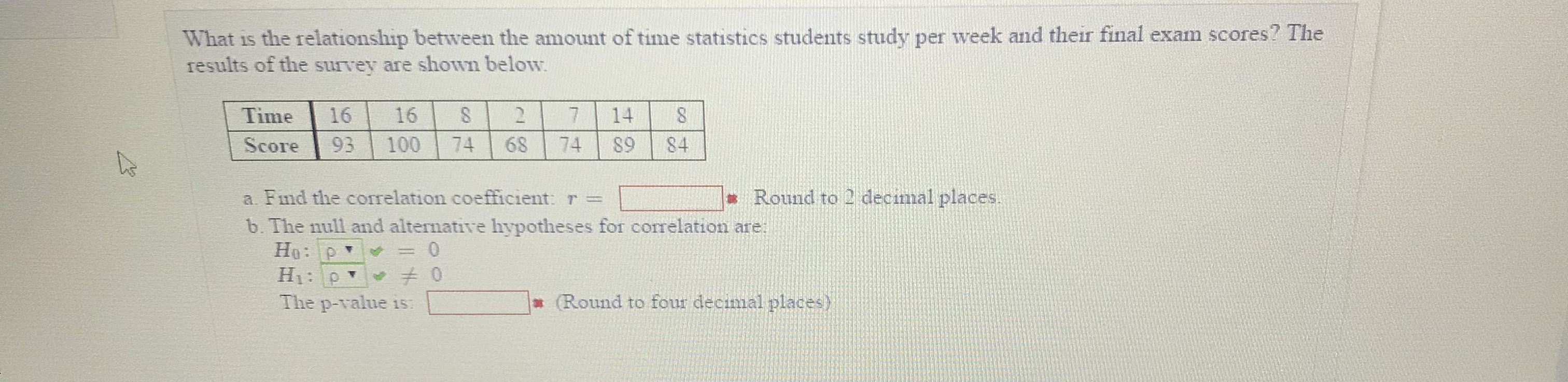What is the relationship between the amount of time statistics students study per week and their final exam scores? Theresults of the survey are shown belowTime 1616 27 14 8Score 93 100 74 68 74 S9 84Round to 2 decimal placesa. Find the correlation coefficient r =b. The null and alternative hypotheses for corelation are:The p-value isRound to four decimal places)

Questionhelp_outlineImage TranscriptioncloseWhat is the relationship between the amount of time statistics students study per week and their final exam scores? The results of the survey are shown below Time 1616 27 14 8 Score 93 100 74 68 74 S9 84 Round to 2 decimal places a. Find the correlation coefficient r = b. The null and alternative hypotheses for corelation are: The p-value is Round to four decimal places) fullscreen
Step 1

a.Correlation coefficient(r):

Step 2

By using Excel formula, “=CORREL(Array1, Array2)”, the correlation between time and score is 0.94.

Step 3

b.

The null and the alternati...

Want to see the full answer?

See Solution

Want to see this answer and more?

Our solutions are written by experts, many with advanced degrees, and available 24/7

See Solution
Tagged in

Statistics